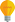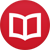# Functional (unified) notation for chord progressions

by Jakub MarianTip: See my list of theMost Common Mistakes in English. It will teach you how to avoid mis­takes with com­mas, pre­pos­i­tions, ir­reg­u­lar verbs, and much more.

The functional notation for chord progressions is an extension of the traditional notation which is fully compatible with it (you won’t have to learn any new symbols if all you want to do is to play a given chord progression on an instrument). It is a notation I have developed in order to speed up the process of learning to understand harmonic progressions in music.

In order to understand the notation, it is first necessary to turn our attention to the structure of the diatonic scale (i.e. the major or the natural minor scale). Let me use the C major/A minor scale as an example. Most people think about A B C D E F G as about the order of the tones of the scale, and indeed, this is the order we usually use when thinking about melodies.

However, there is another, perhaps even more important order of the scale. All the tones of the scale can be ordered so that each step represents a perfect fifth (this order is what musicians often call “the cycle of fifths”). In the case of C major/A minor, it is:

F C G D A E B

This gives us a lot of insight into the structure of the scale. The scale can be decomposed into two important building blocks:

F C G (major subdominant – major tonic – major dominant)
D A E (minor subdominant – minor tonic – minor dominant)

Using just these blocks, we can form the most important chord progression in western music, the I – IV – V – I progression (tonic – subdominant – dominant – tonic), in both major and minor scales. In C major, the progression is: CFGC. In A minor, it is: AmDmEAm.

Most harmonic progressions in western music can be thought of as a combination of these six basic elements divided into two blocks. For example, the ubiquitous I – vi – ii – V – I (e.g. CAmDmGC) is, in fact, just major tonic – minor tonic – minor subdominant – major dominant – major tonic.

Chord progressions often roam freely between the major part and the minor part of a scale. The most common progression in jazz music, the I – ii – V – I progression, is nothing else then tonic – subdominant – dominant – tonic with the subdominant borrowed from the minor part of the scale.

However, the classical Roman numeral notation completely ignores this relationship. Major scales and minor scales are treated as two separate scales, so the minor block in a major scale is denoted ii – vi – iii, and the major block in a minor scale is denoted VI – III – VII. It would be much easier to give every chord of the diatonic scale a single name and acknowledge the fact that the same chord can be used in two different contexts, and this is what I am going to do in this article

## The unified notation

The harmonic functions of the C major/A minor scale will be called as follows:

 F C G D A E B S M D s m d $\sigma$

that is:

S: major subdominant
M: major tonic
D: major dominant
s: minor subdominant
m: minor tonic
d: minor dominant
$\sigma$: diminished subdominant

I use the Greek letter $\sigma$ (sigma) to denote the diminished subdominant, as there is not a third variant of the letter “S” in the Latin alphabet.

## How to remember harmonic functions using this system

The fact that each major/minor scale combination consists of two blocks of the form X Y Z means that, once you memorize all these blocks (and there are essentially only 7 of those), you will be able to reconstruct the harmonic functions of pretty much any scale easily. The blocks are as follows:

B$\flat$ F C
F C G
C G D
G D A
D A E
A E B
E B F$\sharp$

The best way to think about scales is to use pairs of notes for their names (major/minor), e.g. C/A, G/E, D/B. For instance, the major block (subdominant – tonic – dominant) of the D/B scale is G D A, and the minor block is E B F$\sharp$.

If the central letter has an accidental, you just apply the same accidental to the surrounding letters, so a block around D$\sharp$ would be G$\sharp$ D$\sharp$ A$\sharp$. The $\sigma$ has to be memorized separately, but it is luckily just the next letter (sometimes with an accidental) in alphabetic order after the letter denoting the minor tonic, e.g. B in the C/A scale.

## Chord progressions in the unified notation

Our new notation allows us to write chord progressions in a major/minor-independent way. For example, I – IV – V – vi in a major scale and III – VI – VII – i in a minor scale would both be written as

M S D m

(I prefer to omit the dashes). The dichotomy of the traditional notation makes it hard to quickly construct harmonic progressions in your head, which is a skill needed for improvisation. Sure, the traditional notation is somewhat more precise, but this precision is not really needed in practice.

When you improvise a chord progression, there is simply not enough time to analyse it carefully. You have to rely mostly on your intuition, which is guided (in my opinion) mainly by dividing chords into three major categories: tonic, subdominant, and dominant. Whether the chord is in the major block or the minor block is often secondary, and chords from one block can often be used in place of chords from the other block without really damaging the progression.

## The minor dominant and secondary dominants

d, the minor dominant (which is a minor chord by default), has a very mellow sound that does not pull the progression towards the tonic m in the same way D pulls towards M. That’s why it is often replaced by its “majorized” version; by a major triad or a dominant seventh chord.

To indicate this, we shall use the letter $Δ$ (capital delta), to avoid collision with capital D for the major dominant. For example, i – iv – v – i would be m s d m, whereas i – iv – V$^7$ – i would be m s $Δ\!^7$ m.

Dominant chords are often inserted into places where they “don’t belong” to embellish chord progressions. You can imagine that the chord you are about to play is the tonic and insert the corresponding dominant before it. For example, instead of playing I – ii – V$^7$ – I (e.g. CDmG$^7$ – C), you can play I – V$^7$/ii – ii – V$^7$ – I (CA$^7$ – DmG$^7$ – C). This V$^7$/ii (A$^7$) chord is called a secondary dominant.

In the functional notation, secondary dominants will be denoted just like normal dominants, just with a little $\times$ in the subscript. The $\times$ means “look for the next chord; this will be your tonic”. For instance, the progression I – V$^7$/ii – ii – V$^7$ – I would be:

M $\Delta^{7}_\times$ s D$^7$ M

We use the delta here because the dominant leads to a minor chord. When it leads to a major chord, as in the I – V$^7$/V – V$^7$ – I progression, we use D instead:

M D$^7_{\times}$ D$^7$ M

Secondary dominants can also form longer chains, e.g. I – V$^7$/ii – V$^7$/V – V$^7$ – I:

M $\Delta^{7}_\times$ D$^{7}_{\times}$ D$^7$ M

By the way, I have written several educational ebooks. If you get a copy, you can learn new things and support this website at the same time—why don’t you check them out?